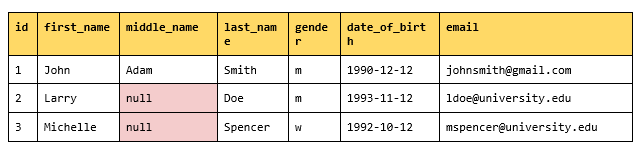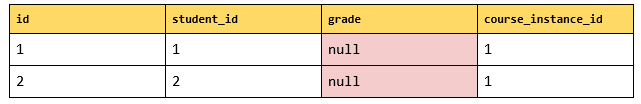# How to Tackle SQL NULLs: The NULLIF FunctionUsually we try to avoid `NULL`s, but sometimes we need to have a `NULL` instead of an actual value. When this need pops up, a helpful but little-known SQL function called `NULLIF` makes it possible. This post will introduce you to `NULLIF` and demonstrate its use in two example cases.

In the article How to Tackle NULLS: The COALESCE Function , we got to know the `COALESCE` function. It returns the first not-null expression. The `NULLIF` function works differently. It accepts two parameters and returns a `NULL` if value1 equals value2. Otherwise, the function returns value1.

The syntax for the `NULLIF` function is:

`NULLIF(value1, value2)`

For example:

```SELECT NULLIF(1, 1);
NULL ```

and

```SELECT NULLIF(1, 0);
1```

This is sugar syntax for the following `CASE` statement:

```CASE
WHEN value1 = value2
THEN NULL
ELSE value1
END```

Now, let’s take a look at some usage examples for the `NULLIF` function.

### Use Case 1: Avoiding “Division by Zero” Errors

Business rules should never be faced with an illegal math function, but it does sometimes happen. For example, we may unintentionally try to divide a value by zero. Suppose we try to calculate the male-female ratio between students registered for a course. What problematic thing might happen here?

Let’s delve into this, starting with the database model shown below. For our query we will need the `student` and `student_courses` tables.

student tablestudent_courses tableSo, let’s do some SQL:

``` WITH
male_student AS (
SELECT COUNT(*) AS male_count
FROM student s
JOIN student_courses c
ON s.id = c.student_id
WHERE gender = 'm'
AND course_instance_id = 1),
female_student AS (
SELECT COUNT(*) AS female_count
FROM student s
JOIN student_courses c
ON s.id = c.student_id
WHERE gender = 'w'
AND course_instance_id = 1)

SELECT male_count/female_count
FROM male_student, female_student
```

Result: ERROR

Upon execution, the above query fails and issues a “division by zero” error when it tries to calculate the male-to-female ratio for the `course_instance_id = 1`.

It happened that the `female_count` in the chosen course equals “0”, so the `SELECT` statement will return a “division by zero” error. To avoid this, we can replace the zero with another value. The best solution is to paste in a `NULL`. There are two ways to do this:  via the `CASE WHEN THEN` expression or the `NULLIF` function.

For the `NULLIF` function, the construction is:

`NULLIF(female_count, 0)`

This will return a `NULL` if the `female_count` equals “0”. If the values aren’t equal, the `female_count` will be returned. In our case, the modification in the `SELECT` statement looks as follows:

```...
...
SELECT male_count/NULLIF(female_count, 0)
FROM male_student, female_student```

Result: NULL

The result will be a `NULL`. Now we can use the `COALESCE` function to replace the final value of the ration (that is currently `NULL`) with a more precise value like “0”.

```...
...
SELECT COALESCE(male_count/NULLIF(female_count, 0), 0)
FROM male_student, female_student```

Result: 0

### Use Case 2: Using NULLIF with Aggregate Functions

The `NULLIF` function can be used when a database contains special non-NULL values that we want to treat as `NULL`s. This is particularly useful for aggregate functions. For example, suppose you need to get the number of records where grades don’t equal zero (the total number of final grades for all courses). Take a look at the table below. In this case, the students that don’t have a final grade yet have a “0” in the `grade` column.

student_courses tableThe query for this is:

```SELECT COUNT(*)
FROM student_courses
Or we can use the `NULLIF` function and skip the `WHERE` clause:
```SELECT COUNT(NULLIF(grade, 0))
So there are times when we actually want `NULL`s? It seems extraordinary, but when you use `NULLIF` it can be quite convenient to have them. With this handy SQL function, we can treat certain values as `NULL` for the sake of better computation.